# Solving problems with path dependent materials

Home » Knowledge Base » General » Materials » Solving problems with path dependent materials

Most nonlinear material models, like the plasticity model, are described by ordinary di erential equations,where Σ and H are the differential equations, h is a vector of history variables (e.g., equivalent plastic strain), and ε is the appropriate strain rate. The di erential equations are shown as depending on the strain rate, and not the strain, to emphasize the dependence of the stress rate through the unknown velocity, ε = Bv. These equations are integrated forward in time at each Gauss point in each element using radial return.In these equations, &SigmaI and HI are the numerical integration methods for advancing the solution over the time increment Δ t. The strain increment across the step is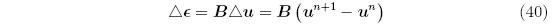and similarly,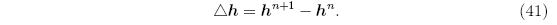In all the discussions about solving nonlinear equations, the current value of a function is calculated using the current value of the solution variables, i.e, fi = f(ui) and fi = u(ui). The path dependence of the material response through its di erential equations introduces a small but important point about how the residual vector should be actually evaluated. A simple model problem limited to small strains illustrates the issues. Assume that for a one dimensional problem, the di erential equation for the stress is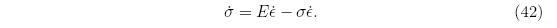The rst term on the right hand side is linear elasticity, expressed in a rate form, and the second term is similar to the one that arises in the Truesdell stress rate. Using forward Euler integration, the stress is updated with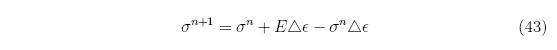The increment in displacement across the step at iteration i, Δ ui, is the sum of the i contributions from the previous iterations, δ uk,The strain increment across the time step for iteration i is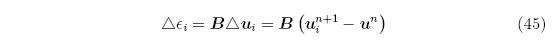and, therefore, the strain increment is the sum of the individual increments for each iteration,A niave implementation of an iterative nonlinear solver would update the stress each iteration withSuppose that the iterative solver converges in two iterations, giving the strain increment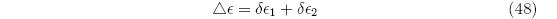or, alternatively,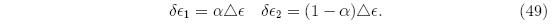Starting with an initial stress σ 0 (and dropping the superscript for the time step), the stress after the first iteration isThe final stress is then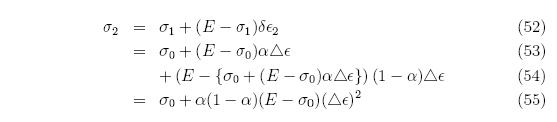The nal stress clearly depends on how the strain increment is partitioned between the two iterations, and achieves a maximum at α = 1/2. Small changes in the iteration strategy can therefore alter the final stress even if the final displacement solution remains the same.

The stress update is made independent of the iterations by always updating the stress with the current strain increment and the stress and history vector from the previous step. In this example, σ 1 remains unchanged from Equation 50, while the nal stress becomes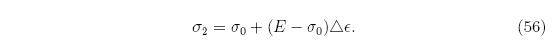More generally, using the notation in Equations 38 and 39, the correct stress and history vector updates within a nonlinear equation solver areNote that during the iteration, the solution at n and n+1 are referenced. Keeping a complete solution state, 1 through n + 1, for every step requires a prohibitive amount of memory, so that only the last two states are kept: the last converged state, n, and the state that is being iterated on, n + 1. After the iterative solution converges, the solution from n + 1 is copied to the storage previously used for state n, the displacement increment over the step, Δ u, is set to zero, and time is advanced t = t + Δ t. The overall solution algorithm is now ready to solve for the solution at n + 2.

djb 2005